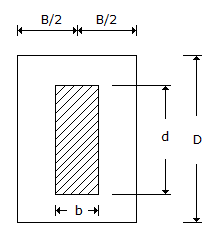# Civil Engineering - UPSC Civil Service Exam Questions

31.

If the stress on the cross-section of a circular short column of diameter D is to be wholly compressive, the load should be applied within a concentric circle of diameter

 A. D/2 B. D/8 C. D/4 D. D/6

Explanation:

No answer description available for this question. Let us discuss.

32.

What does higher standard deviation imply in cost analysis ?

 A. Higher uncertainty B. Lower uncertainty C. Nothing to do with uncertainty D. Extra costs are likely

Explanation:

No answer description available for this question. Let us discuss.

33.

Consider the following statements :
The disease of dry not in timber is caused by :
1. complete submergence in water.
2. alternate wet and dry condition.
3. lack of ventilation.
Which of the statements given above is/are correct ?

 A. 1 only B. 3 only C. 2 only D. 2 and 3

Explanation:

No answer description available for this question. Let us discuss.

34.

A section of a flitched beam is shown in the given figure. The main role of the steel plate placed between the two timber sections is toA. increase the axial resistance of the section B. increase and density C. increase shear and bending resistance of the section D. avoid development of shear stress in the two timber sections

Explanation:

No answer description available for this question. Let us discuss.

35.

A velocity field with no components in the y and z directions is given by V = 6 + 2xy +t2
The acceleration along the x-direction at a point (3, 1, 2), at time 2, is

 A. 8 units B. 16 units C. 28 units D. 36 units